【Stanford CNN课程笔记】5. 神经网络解读1 几种常见的激活函数

2. 建模神经元

2.1 神经元的激活和连接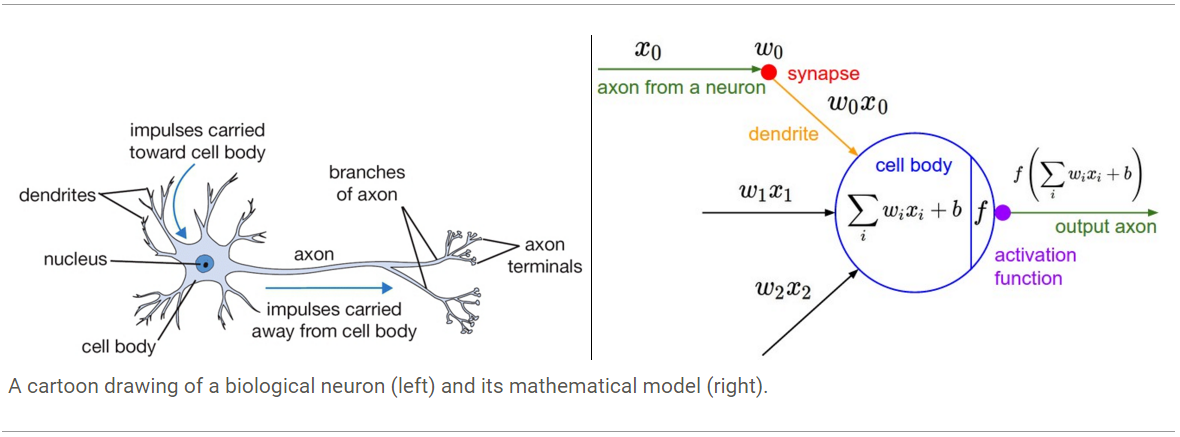class Neuron(object):
# ...
def forward(inputs):
""" assume inputs and weights are 1-D numpy arrays and bias is a number """
cell_body_sum = np.sum(inputs * self.weights) + self.bias
firing_rate = 1.0 / (1.0 + math.exp(-cell_body_sum)) # sigmoid activation function
return firing_rate

2.2 一个神经元就是一个线性分类器

Binary Softmax classifier. 比如说，我们可以把σ(iwixi+b)$\sigma(\sum_iw_ix_i+b)$看成是某类的概率P(yi=1|xi;w)$P(y_i=1|x_i;w)$，那么另一类的概率则是P(yi=0|xi;w)=1P(yi=1|xi;w)$P(y_i=0|x_i;w)=1-P(y_i=1|x_i;w)$，因为对于二值分类器而言两类的概率相加应为1。然后我们再通过在线性分类器那一章见过的交叉熵loss对score的好坏进行量化，这就是一个二值softmax分类器了（也叫逻辑回归）。因为sigmoid function会把只限定于0-1之间，分类器可以通过判断上述概率是否大于0.5来进行分类。
Binary SVM classifier. 我们还可以选择边界最大化的hinge loss来衡量神经元输出的好坏，那么此时就变成了一个二值SVM分类器了。

2.3 几种常见的激活函数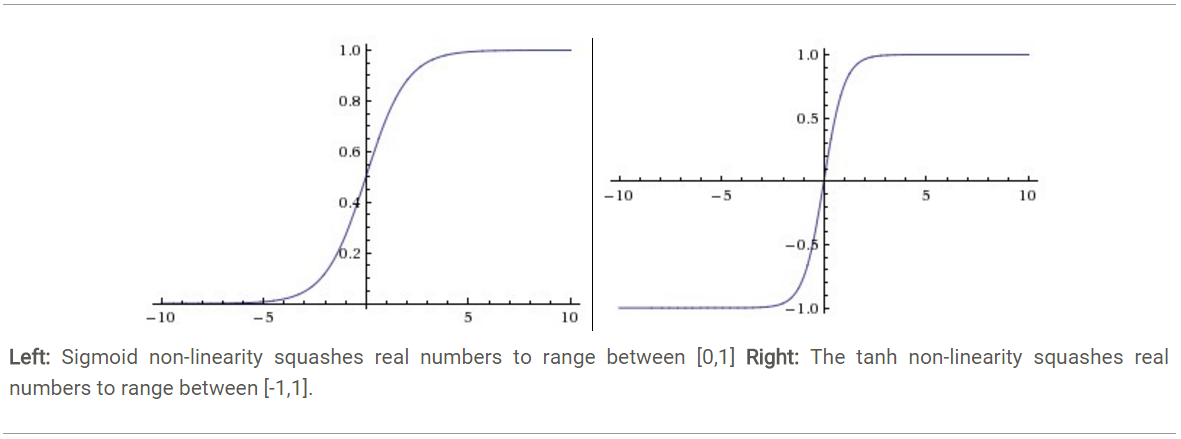Sigmoid. Sigmoid 非线性激活函数的形式是σ(x)=1/(1+ex)$\sigma(x)=1/(1+e_{-x})$，其图形如上图左所示。之前我们说过，sigmoid函数输入一个实值的数，然后将其压缩到0~1的范围内。特别地，大的负数被映射成0，大的正数被映射成1。sigmoid function在历史上流行过一段时间因为它能够很好的表达“激活”的意思，未激活就是0，完全饱和的激活则是1。而现在sigmoid已经不怎么常用了，主要是因为它有两个缺点:

• Sigmoid outputs are not zero-centered. Sigmoid 的输出不是0均值的，这是我们不希望的，因为这会导致后层的神经元的输入是非0均值的信号，这会对梯度产生影响：假设后层神经元的输入都为正(e.g. x>0 elementwise in f=wTx+b$f=w_Tx+b$),那么对w求局部梯度则都为正，这样在反向传播的过程中w要么都往正方向更新，要么都往负方向更新，导致有一种捆绑的效果，使得收敛缓慢。

Tanh. Tanh和Sigmoid是有异曲同工之妙的，它的图形如上图右所示，不同的是它把实值得输入压缩到-1~1的范围，因此它基本是0均值的，也就解决了上述Sigmoid缺点中的第二个，所以实际中tanh会比sigmoid更常用。但是它还是存在梯度饱和的问题。Tanh是sigmoid的变形：tanh(x)=2σ(2x)1$tanh(x)=2\sigma(2x)-1$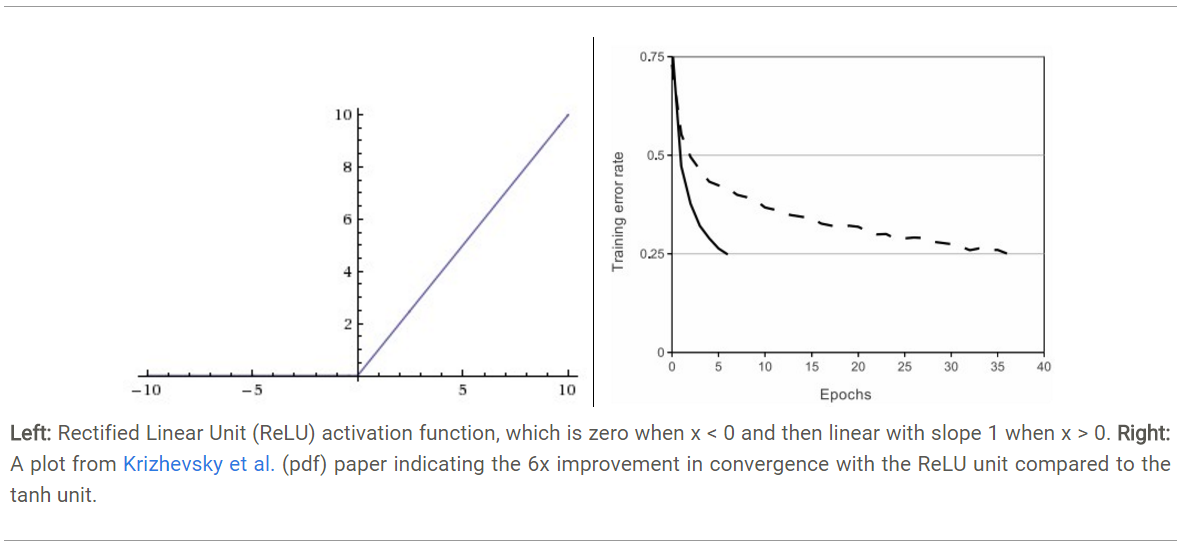ReLU. 近年来，ReLU 变的越来越受欢迎。它的数学表达式是： f(x)=max(0,x)。很显然，从上图左可以看出，输入信号
<0时，输出为0，>0时，输出等于输入。ReLU的优缺点如下：

• 优点1：Krizhevsky et al. 发现使用 ReLU 得到的SGD的收敛速度会比 sigmoid/tanh 快很多(如上图右)。有人说这是因为它是linear，而且梯度不会饱和
• 优点2：相比于 sigmoid/tanh需要计算指数等，计算复杂度高，ReLU 只需要一个阈值就可以得到激活值。
• 缺点1： ReLU在训练的时候很”脆弱”，一不小心有可能导致神经元”坏死”。举个例子：由于ReLU在x<0时梯度为0，这样就导致负的梯度在这个ReLU被置零，而且这个神经元有可能再也不会被任何数据激活。如果这个情况发生了，那么这个神经元之后的梯度就永远是0了，也就是ReLU神经元坏死了，不再对任何数据有所响应。实际操作中，如果你的learning rate 很大，那么很有可能你网络中的40%的神经元都坏死了。 当然，如果你设置了一个合适的较小的learning rate，这个问题发生的情况其实也不会太频繁。

Leaky ReLU. Leaky ReLUs 就是用来解决ReLU坏死的问题的。和ReLU不同，当x<0时，它的值不再是0，而是一个较小斜率(如0.01等)的函数。也就是说f(x)=1(x<0)(ax)+1(x>=0)(x),其中a是一个很小的常数。这样，既修正了数据分布，又保留了一些负轴的值，使得负轴信息不会全部丢失。关于Leaky ReLU 的效果，众说纷纭，没有清晰的定论。有些人做了实验发现 Leaky ReLU 表现的很好;有些实验则证明并不是这样。
- PReLU. 对于 Leaky ReLU 中的a，通常都是通过先验知识人工赋值的。然而可以观察到，损失函数对a的导数我们是可以求得的，可不可以将它作为一个参数进行训练呢? Kaiming He 2015的论文《Delving Deep into Rectifiers: Surpassing Human-Level Performance on ImageNet Classification》指出，不仅可以训练，而且效果更好。原文说使用了Parametric ReLU后，最终效果比不用提高了1.03%.
-Randomized Leaky ReLU. Randomized Leaky ReLU 是 leaky ReLU 的random 版本, 其核心思想就是，在训练过程中，a是从一个高斯分布中随机出来的，然后再在测试过程中进行修正。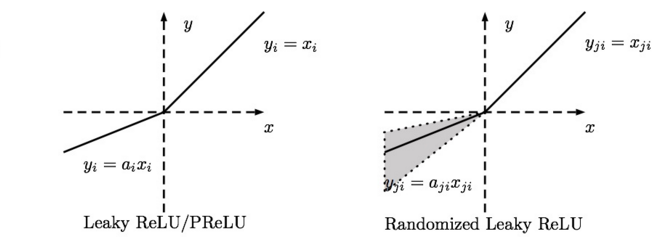Maxout. Maxout的形式是f(x)=max(w_1^Tx+b_1,w_2^Tx+b_2)，它最早出现在ICML2013上，作者Goodfellow将maxout和dropout结合后，号称在MNIST, CIFAR-10, CIFAR-100, SVHN这4个数据上都取得了start-of-art的识别率。可以看出ReLU 和 Leaky ReLU 都是Maxout的一个变形，所以Maxout 具有 ReLU 的优点（如：计算简单，不会 saturation），同时又没有 ReLU 的一些缺点 （如：容易饱和）。不过呢Maxout相当于把每个神经元的参数都double了，造成参数增多。
Maxout的拟合能力非常强，它可以拟合任意的的凸函数。作者从数学的角度上也证明了这个结论，即只需2个maxout节点就可以拟合任意的凸函数了(相减)，前提是”隐含层”节点的个数可以任意多。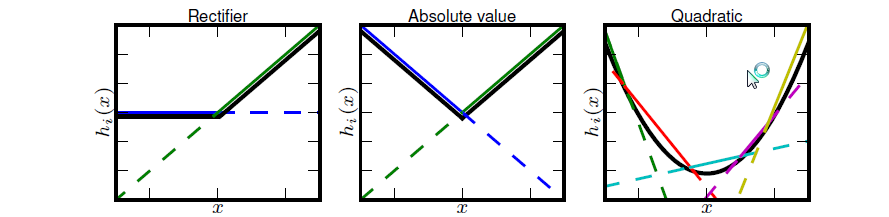How to choose a activation function? 怎么选择激活函数呢?
我觉得这种问题不可能有定论的吧，只能说是个人建议。
如果你使用 ReLU，那么一定要小心设置 learning rate，而且要注意不要让你的网络出现很多坏死的 神经元，如果这个问题不好解决，那么可以试试 Leaky ReLU、PReLU 或者 Maxout.
友情提醒：最好不要用 sigmoid，你可以试试 tanh，不过可以预期它的效果会比不上 ReLU 和 Maxout.
还有，通常来说，很少会把各种激活函数串起来在一个网络中使用的。

3. 神经网络结构

3.1 逐层构建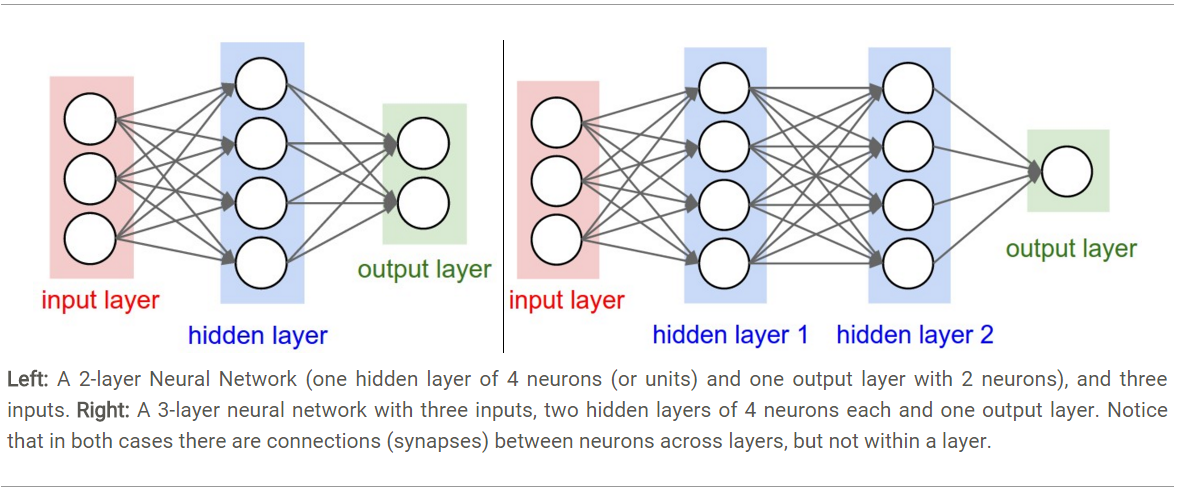- 上图左的网络共包含（4+2=6）个神经元（不包括输入层），参数则有[3*4]+[4*2]=20个weights还有[4+2=6]个bias，也就是总共26个可学习的参数。
- 上图右的网络共包含（4+4+1=9）个神经元，参数则有[3*4]+[4*4]+[4*1]=12+16+4=32个weights还有[4+4+1=9]个bias，也就是总共41个可学习的参数。

3.2 例子：前向传播的计算

# forward-pass of a 3-layer neural network:
f = lambda x: 1.0/(1.0 + np.exp(-x)) # activation function (use sigmoid)
x = np.random.randn(3, 1) # random input vector of three numbers (3x1)
h1 = f(np.dot(W1, x) + b1) # calculate first hidden layer activations (4x1)
h2 = f(np.dot(W2, h1) + b2) # calculate second hidden layer activations (4x1)
out = np.dot(W3, h2) + b3 # output neuron (1x1)

The forward pass of a fully-connected layer corresponds to one matrix multiplication followed by a bias offset and an activation function.

3.4 如何设置神经网络的层数和神经元数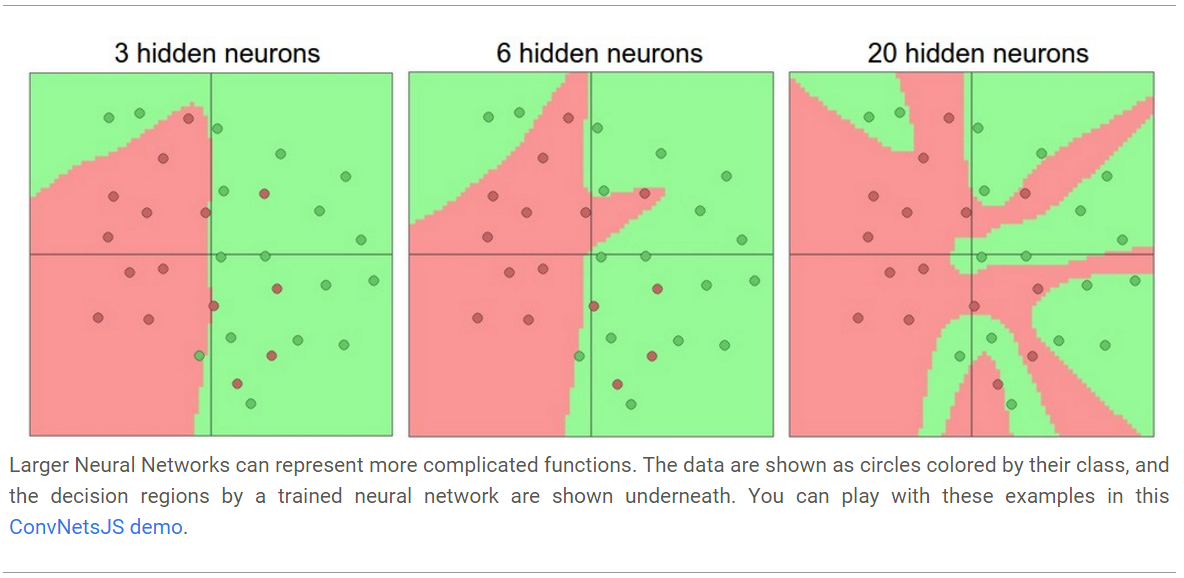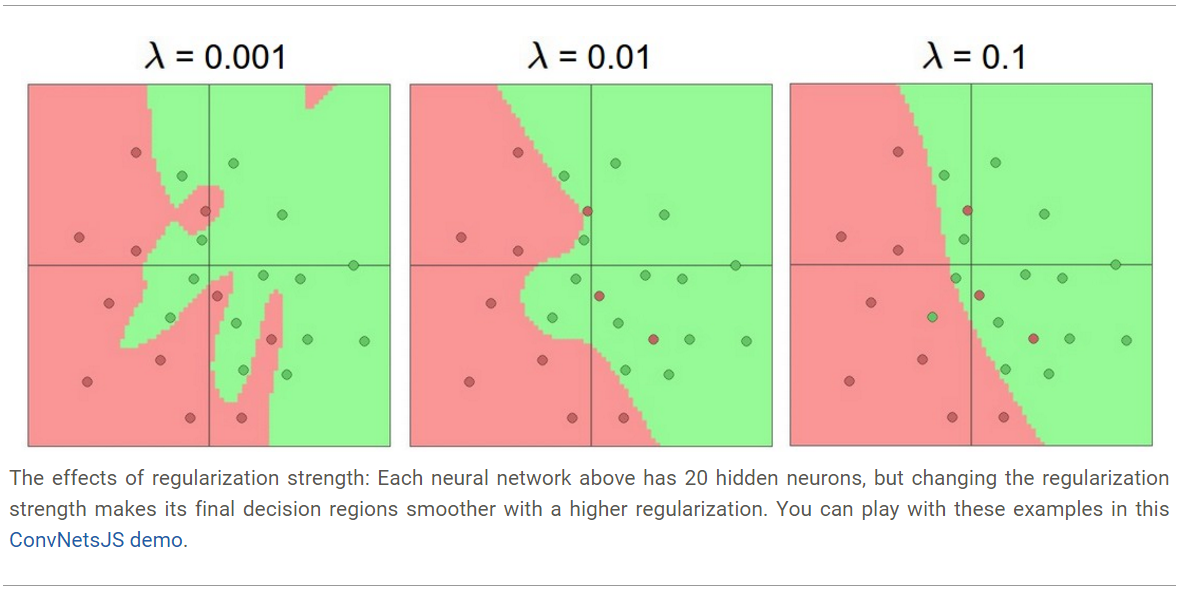4. 总结

- 我们粗略地介绍了生物的神经元
- 我们讨论了几种实际使用的激活函数，其中ReLU是最常用的选择
- 我们介绍了神经网络，其中神经元是通过全连接层来组织的，全连接层中相邻层的神经元两两连接，同一层的神经元互相不连接
- 我们发现这种层级的结构使得神经网络只要进行矩阵乘法和激活函数就可以计算了
- 我们发现神经网络是万能逼急器，但是我们也说了这种特性并不能表示我们不需要go deeper。
- 我们讨论了大型的神经网络总是会比小型神经网络效果要好，但是这也导致它们有可能会过拟合，因此我们需要选用恰当的正则方法来弥补这一缺点，在后面我们会见到更多形式的正则（如dropout等）。